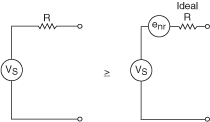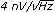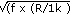# Noise

NI Digital Multimeters Help (NI-DMM 18.1)

Edition Date: March 2018

Part Number: 370384V-01

»View Product InfoDownload Help (Windows Only)

Noise in a measurement can originate from the instrument taking the measurement or from an interfering signal passing through the instrument and causing measurement instability. When considering noise, you need to know the measurement bandwidth because it sets the bounds for how you can manage the noise. You can decrease the measurement bandwidth by increasing the aperture of the measurement or by averaging the measurement.

Noise in the system is a common challenge in designing measurement systems. Noise sources in the environment can be electrostatically or inductively coupled in from the powerline; therefore, most DMMs specify noise rejection at line frequencies of 50 Hz or 60 Hz. The rejection at 400 Hz is, at a minimum, as good as the rejection at 50 Hz because the aperture time for 50 Hz also eliminates 400 Hz components. For more information about how to configure the NI 4065 and NI 4070/4071/4072 for optimum normal-mode rejection ratio (NMRR), refer to DC Noise Rejection.

### Johnson Noise of Resistors

A commonly overlooked source of noise in precision instrumentation is the source noise resistance, as shown in the following figure.Even a simple fixed-value resistor is noisy. The inherent noise generated by a non-ideal resistor is represented by a noise voltage source (enr) in series with the ideal, noise-free resistance. This noise results from random motion of thermally generated electrons in the material. This signal is usually called Johnson noise. The Johnson's equation normalized to 1 kΩ results in the following equation:

Vn = 4[√(R/1 kΩ)][(nV/√(Hz))]

enr = √(4kTRf)

This noise, which is present in every resistor at common laboratory temperatures, is a function of temperature, the value of the resistance, and the bandwidth of the measurement. The noise is defined as:

enr = 1.28*10–10*√(Rf) rms

You can convert this equation to:

enr = 8.45 * 10–10 * √(Rf) p–p

where

R =resistance being measured

f = noise bandwidth of the measurement

This equation assumes you are using ideal resistor elements that exhibit white noise. The white noise is assumed to be a Gaussian distribution. Some resistors, such as certain carbon film resistors, can generate noise from other mechanisms when current is passed through them. Metal foil and wire wound resistors approach this theoretical limit.

As a point of reference to simplify the calculation, a 1 kΩ resistor hasrms noise density (1 Hz bandwidth). You can scale this value to get to any noise level given any resistor or frequency by multiplying it by. For example, a 100 kΩ resistor in a 100 Hz bandwidth has a noise of:

enr = 4 nV√(Hz) * √(100 * (100 kΩ / 1 kΩ))

enr = 400 nVrms

If the DMM is digitizing at 1 kS/s, the measurement bandwidth is 1 kHz and the effective noise is:

enr = 4 nV√(Hz) * √(1,000 * (100 kΩ / 1 kΩ))

enr = 4 nV x 316

enr = 1.26 µVrms or 8.3 µVp-p

Thus, the source resistance limits the noise floor of the measurement over a 1 kHz bandwidth to 8.3 µVp-p.